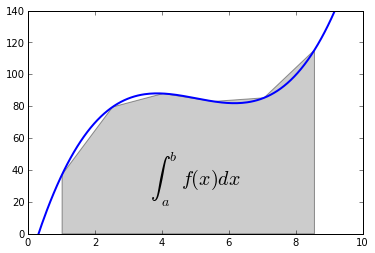Basic numerical integration: the trapezoid rule¶

A simple illustration of the trapezoid rule for definite integration:

$$\int_{a}^{b} f(x)\, dx \approx \frac{1}{2} \sum_{k=1}^{N} \left( x_{k} - x_{k-1} \right) \left( f(x_{k}) + f(x_{k-1}) \right).$$

First, we define a simple function and sample it between 0 and 10 at 200 points

In :
%pylab inline
Welcome to pylab, a matplotlib-based Python environment [backend: module://IPython.zmq.pylab.backend_inline].
In :
def f(x):
return (x-3)*(x-5)*(x-7)+85

x = linspace(0, 10, 200)
y = f(x)

Choose a region to integrate over and take only a few points in that region

In :
a, b = 1, 9
xint = x[logical_and(x>=a, x<=b)][::30]
yint = y[logical_and(x>=a, x<=b)][::30]

Plot both the function and the area below it in the trapezoid approximation

In :
plot(x, y, lw=2)
axis([0, 10, 0, 140])
fill_between(xint, 0, yint, facecolor='gray', alpha=0.4)
text(0.5 * (a + b), 30,r"$\int_a^b f(x)dx$", horizontalalignment='center', fontsize=20);Compute the integral both at high accuracy and with the trapezoid approximation

In :